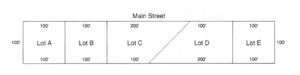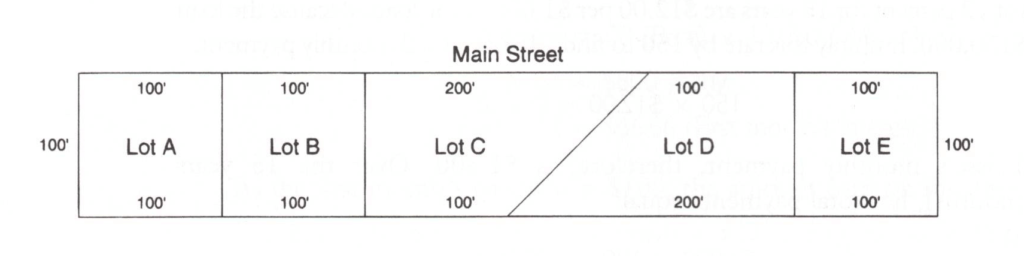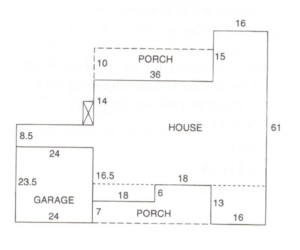# Học Thi Real Estate License ở California: Practice Real Estate Mathematics

1.

A three-strand wire fence around a square 40-acre parcel would require how much wire?

2.

A woman purchased a property for $15,000 with$2,000 down and no payments or interest for one year. At the end of the year, she sold the property at double the purchase price. Each dollar of the original investment is now worth:

3.

A 10-acre rectangular lot on High Street sold for $60,000. Assuming a depth of 300', the price paid per front foot is: 4. Jay sells a lot for 20 percent more than he paid for it and invests the entire proceeds in an investment yielding nine percent per year. His monthly return on his investment is$320. What did Jay originally pay for the lot?

5.

If the lots in the figure sell for $10 per front foot on Main Street, Lot D would sell for:6. A property was listed to give the owner$41,000 after the six percent commission was paid. It was listed at:

7.

In a cubic yard there are:

8.

A road runs along the south boundary of the SW 1/4 of the SW 1/4 of a section. If the road contains two acres in area, the width of the road would be:

9.

Oliver sells his house for $14,900. This is 18 percent more than he paid for it. Oliver paid: 10. To find the square footage of a square 40 arce parcel: 11. The maximum number of 50' x 75' lots that can be made from one acre would be: 12. A sales manager received$3,500 per month plus 0.1 percent of office sales. For March, she received $7,400. This was based on monthly sales of: 13. A mil is expressed as: 14. To find the depth of a rectangular parcel when the width and the total area are known: 15. If Lot C sells for$1 per square foot, it would sell for:16.

Frank buys a $1,200 trust deed at a 25 percent discount. The trust deed requires payments of$104 per month, including interest for one year. Frank's percentage of return for the year on his investment is:

17.

Nate has 10 apartments fully rented at $100 per month. He raises his rent 10 percent and experiences a 10 percent vacancy factor. 18. If the lots in the figure sell for$100 per front foot on Main Street, Lot A would sell for:19.

An investment that would pay $100 per month interest at six percent would require an investment of: 20. In the figure, how many square feet are contained in the living area of the dwelling only?21. In the preceding question, the percentage of profit based on selling price is: 22. A property was purchased for$10,000 and has a gross income of $2,000. The only expense is the owner's interest payment of nine percent on an$8,000 trust deed. The owner's percentage of return on his equity is:

23.

A 2'' x 8'' board 9 feet long would contain  ___ board feet,

24.

You wish to earn $50 per month on a six percent investment. How much would you have to invest? 25. A speculator purchased a home at 25 percent less than list price and later sold it for the original list price. The percentage of profit was: 26. An 80 -acre parcel purchased for$400 per acre is resold in four 20-acre parcels at $1000 per acre. The percentage of profit based on cost is: 27. A man paid off an eight percent term loan for$800. His total interest cost was $42.66. He paid off the loan in: 28. A borrower was required to give the lender a deposit equal to three months' taxes and insurance cost. If the property was 0,024 per dollar of assessment and the yearly insurance cost was$462, what deposit was required?

29.

A man purchased three lots for $17,000. Lot 2 sells for$1,500 more than Lot 1, and Lot 3 sells for $2,000 more than Lot 2. What is the full price of Lot 2? 30. A rectangular piece of property has 200 feet on one side and an apartment building that is worth$193,600, which is the equivalent of $4.40 per square foot for the lot. What is the front footgage? 31. If barberd wire weighs one pound per rod, how many pounds of barbed wire will it take to put a single-strand fence around a section? 32. Nine cubic yards of concrete is equal to: 33. Two houses sold together for$49,911. If one was valued $3,176 more than the other, what did the more expensive home sell for? 34. A 5 foot fence will be built around the perimeter of a 50' x 120' rectangular lot. If the fence will cost$1.80 per linear foot for labor and $.40 per square foot for material, what will be the cost of the fence? 35. A property owner had an assessed value of$10,000 on his home, with taxes at $8 per$100 assessed valuation, making his taxes $800. The next year, the tax rate went up to$9 per $100, making his taxes$900. The assessed value:

36.

Claude wishes to pour a concrete patio 40' x 10' and four inches thick. How many cubic yards of concrete are needed?

37.

Seller A offers the S 1/2 of the NW 1/4 of the NE 1/4 of Section 21 for sale through Broker B at a list price of $350 per acre. Broker B presents Buyer C's offer of$6,100 to Seller A. Seller A accepts Buyer C's offer provided that the buyer pays the broker 10 percent commission on the offered price. If the buyer accepts this provision, how much less than the original list price did the buyer actually pay?

38.

If thw buyer's down payment is $12,000 and the property has a net income of$8,300, the buyer's percentage of return on the down payment is:

39.

A rectangular parcel 422 feet wide contains 8.3 acres. Its depth is:

40.

An investor purchased two lots at $6,000 each and divided them into three lots, which he sold for$4,800 each. His percentage of profit based on cost was:

41.

A lot is assessed at 25 percent of its $7,500 market value. The tax rate is .02392 per dollar of valuation. Taxes would be: 42. The easiest way to compute one month's interest at 7.2 percent would be to multiply the principal by: 43. Mr. Jones had sales of$25,000. His markup was 50 percent of his cost. The merchandise cost him:

44.

A man owned a rectangular property with an area of 3/4 of an acre and a depth of 110 feet. He purchased the adjoining property, which was 2/3 the size of his own with the same depth, for $1,400. Putting them together into rectangular lots of 82.5' x 110' each, he sold the lots for$750 each, which was 50 percent over his cost. How much did he pay for his original property?

45.

Which of the following possible transaction will result in the greatest net to a seller?

Sale Price       Commission        Closing Costs
a. $640,000 5%$13,800
b. $638,000 6 1/2%$10,200
c. $637,500 6 .4%$3,100
c. $628,000 4.5%$2,450

46.

The last month's interest on a six percent loan was $739.12. What was the loan balance? 47. The interest paid last month on a six percent loan was$4,900. The balance on the loan was:

48.

A $10,000 loan requires payments of$100 per month plus interest. As to the monthly payments, the amount of money applying to:

49.

A woman borrowed $5,000 for one year with six percent prepaid interest. She received: 50. What percentage of a section is 1/90 of a township? 51. A property was purchased for$87,500. The buyer now wishes to sell it. If selling costs  will equal 11 percent of any sales price, how much must the property appreciate in value for the seller to break even?

52.

On a 90' x 90' lot, the building setbacks are 30' from the front, and 10' from the sides, and 20' from rear line. The net building area is:

53.

A board foot of lumber cannot be obtained from a board:

54.

On a 25-year lease at $15,000 a year, a broker gets a commission of seven percent for the first year, five percent for the next four years, three percent for the next 10 years, and one percent for the remainder of the lease. How much commission has the broker earned by the end of the nineteenth year? 55. Lance sells a new note at a four percent discount. He receives$5,200. The face amount of the note is:

56.

The cost-of-living index increased from 100 to 120. What effect does this have the purchasing power of the dollar?

57.

An owner divides a one-acre parcel into four equal rectangular lots, each 200 feet deep. What is  the approximate width of each lot?

58.

Lucy owes $8,100 on a note. Her next monthly interest payment will be$58.32 Annual interest on the note is:

59.

How many acres are there in 580,800 square yards?

60.

If the tax rate is $2.392 per$100 of assessed valuation and a property is assessed at 25 percent of its real value of $47,500, what are the taxes? 61. A 10-arce rectangular parcel is divided into six equal lots, each having a depth of 250'. The width of each lot is approximately how many feet? 62. A road runs along the south boundary of a section. It contains three acres. Its width is: 63. Elena sells her home for$40,000, making a 30 percent profit on what she paid for it:

64.

Lot E has a building setback of 30 feet from each side. The maximum square footage of a two-story building on Lot E would be:65.

Given the amount of interest paid, the rate of interest, and the time, which formula would you use to find the amount of the principal?

66.

A lender agrees to make a 90 percent loan on a home selling for \$220,000 providing the borrower pays loan costs of 1 1/2 percent. What are the loan costs?